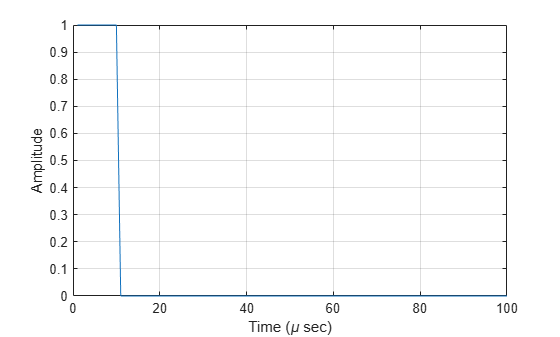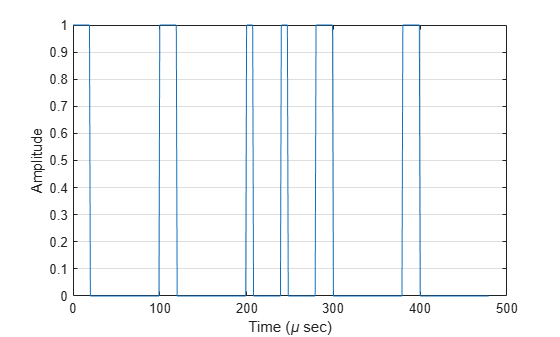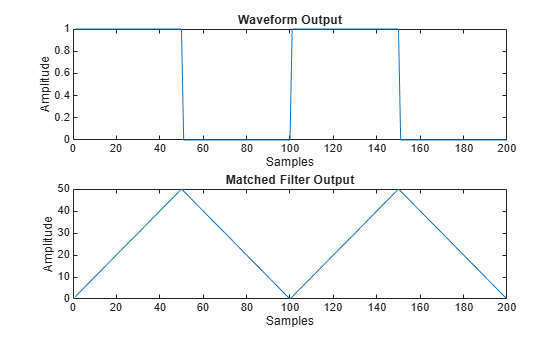step

System object: phased.RectangularWaveform
Package: phased

Samples of rectangular pulse waveform

Syntax

Y = step(sRFM)
Y = step(sRFM,prfidx)
Y = step(sRFM,freqoffset)
[Y,PRF] = step(___)
[Y,COEFF] = step(___)

Description

Note

Starting in R2016b, instead of using the step method to perform the operation defined by the System object™, you can call the object with arguments, as if it were a function. For example, y = step(obj,x) and y = obj(x) perform equivalent operations. When the only argument to the step method is the System object itself, replace y = step(obj) by y = obj().

Y = step(sRFM) returns samples of a rectangular pulse in the column vector Y.

Y = step(sRFM,prfidx), uses the prfidx index to select the PRF from the predefined vector of values specified by the PRF property. This syntax applies when you set the PRFSelectionInputPort property to true.

Y = step(sRFM,freqoffset), uses the freqoffset to generate the waveform with an offset as specified at step time. Use this syntax for cases where the transmit pulse frequency needs to be dynamically updated. This syntax applies when you set the FrequencyOffsetSource property to 'Input port'.

[Y,PRF] = step(___) also returns the current pulse repetition frequency, PRF. To enable this syntax, set the PRFOutputPort property to true and set the OutputFormat property to 'Pulses'.

[Y,COEFF] = step(___) returns the matched filter coefficients, COEFF, for the current pulse. To enable this syntax, set CoefficientsOutputPort to true. COEFF is returned as either an NZ-by-1 vector or an NZ-by-M matrix.

• An NZ-by-1 vector is returned when:

• The object has OutputFormat set to 'Pulses' and NumPulses is equal to 1. NZ is the pulse width.

• The object is configured to generate constant pulse width waveforms (DurationSpecification is set to 'Pulse width' or 'Duty cycle' and PRF has one unique value); and either OutputFormat is set to 'Pulses' and NumPulses is greater than 1, or OutputFormat is set to 'Samples'. For this case, NZ is the pulse width.

• An NZ-by-M matrix is returned when the object generates varying pulse widths (DurationSpecification is set to 'Duty cycle' and PRF has more than one unique value); and either OutputFormat set to 'Pulses' and NumPulses is greater than 1, or OutputFormat is set to 'Samples'. For this case, NZ is the pulse width, and M is the number of sub-pulses, NumSteps.

You can combine optional input and output arguments when their enabling properties are set. Optional inputs and outputs must be listed in the same order as the order of the enabling properties. For example, [Y,PRF,COEFF] = step(sRFM,prfidx,freqoffset).

Note

The object performs an initialization the first time the object is executed. This initialization locks nontunable properties and input specifications, such as dimensions, complexity, and data type of the input data. If you change a nontunable property or an input specification, the System object issues an error. To change nontunable properties or inputs, you must first call the release method to unlock the object.

Examples

expand all

Construct a 10 microseconds rectangular pulse with a pulse repetition interval of 100 microseconds.

Pulsewidth = 10e-6;
PRI = 100e-6;
sRFM = phased.RectangularWaveform('PulseWidth',Pulsewidth,...
'OutputFormat','Pulses','NumPulses',1,...
'SampleRate',1e6,'PRF',1/PRI);
wav = step(sRFM);
plot(wav)
xlabel('Time (\mu sec)')
ylabel('Amplitude')
gridConstruct rectangular waveforms with two pulses each. Set the sample rate to 1 MHz, a pulse width of 50 microseconds, and a duty cycle of 20%. Vary the pulse repetition frequency.

Set the sample rate and PRF. The ratio of sample rate to PRF must be an integer.

fs = 1e6;
PRF = [10000,25000];
waveform = phased.RectangularWaveform('OutputFormat','Pulses','SampleRate',fs,...
'DurationSpecification','Duty Cycle','DutyCycle',.2,...
'PRF',PRF,'NumPulses',2,'PRFSelectionInputPort',true);

Obtain and plot the rectangular waveforms. For the first call to the step method, set the PRF to 10 kHz using the PRF index. For the next call, set the PRF to 25 kHz. For the final call, set the PRF to 10 kHz.

wav = [];
wav1 = waveform(1);
wav = [wav; wav1];
wav1 = waveform(2);
wav = [wav; wav1];
wav1 = waveform(1);
wav = [wav; wav1];
nsamps = size(wav,1);
t = [0:(nsamps-1)]/waveform.SampleRate;
plot(t*1e6,real(wav))
xlabel('Time (\mu sec)')
ylabel('Amplitude')
gridGenerate output samples and matched filter coefficients of a rectangular pulse waveform.

waveform = phased.RectangularWaveform('CoefficientsOutputPort',true, ...
'PRF',[1e4 2e4],'DurationSpecification','Duty cycle','DutyCycle',0.5, ...
'OutputFormat','Pulses','NumPulses',2,'PRFSelectionInputPort',true);
[wav,coeff] = waveform(1);

Create a matched filter that applies the coefficients as an input argument. Use the coefficients when applying the matched filter to the waveform. Plot the waveform and matched filter outputs.

mf = phased.MatchedFilter('CoefficientsSource','Input port');
mfOut = mf(wav,coeff(:,1));
subplot(211),plot(real(wav));
xlabel('Samples'),ylabel('Amplitude'),title('Waveform Output');
subplot(212),plot(abs(mfOut));
xlabel('Samples'),ylabel('Amplitude'),title('Matched Filter Output');